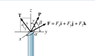# Determine the components of P in the i, j, and k directions.

• anonymous812
In summary, P is a force that is projected along the x axis. The x component of P is zero if alpha is 90 degrees or 270 degrees.

## Homework Statement

As shown, a pole is subjected to three forces: F, P, and T. The force F expressed in Cartesian vector form is F=[5 i+7 j+6 k] N. Force P has magnitude 7.48 N and acts in the direction given by these direction angles: α=143∘, β=57.7∘, and γ=74.5∘ to the x, y, and z axes, respectively. Force T lies within the yz plane, its direction is given by the 3-4-5 triangle shown, and its magnitude is 14 N.
Determine the components of P in the i, j, and k directions.

## Homework Equations

A_x=Acos(α)
cos^2(α)+cos^2(β)+cos^2(λ)=1

## The Attempt at a Solution

Honestly, I'm very confused as to how to approach this problem. I was under the impression that the x component was 0, y component was 8.40 and the z component was 11.2. I solved these by making a 2D plane from the P vector into a 3-4-5 right triangle. 3 on y, 4 on z, and 5 as the hyp.
I would really appreciate a better explanation of how to approach this question..I think I'm making it more difficult than it is.
Thank you!

#### Attachments

•Screen Shot 2013-08-28 at 3.47.09 PM.png
4.8 KB · Views: 1,538
Why arer F and T given if P is the only force to be found? It's not as if P is somehow related to T and/or F.

There are multiple parts to the question.. I'm only asking about the P vector.

anonymous812 said:
1. . I was under the impression that the x component was 0, y component was 8.40 and the z component was 11.2. I solved these by making a 2D plane from the P vector into a 3-4-5 right triangle. 3 on y, 4 on z, and 5 as the hyp.
I would really appreciate a better explanation of how to approach this question..I think I'm making it more difficult than it is.
Thank you!

Taking just the x component of P, the only way that component could be zero is if alpha = 90 (or 270) deg.

Look at P: what is the projection of P along the x axis?
Hint: cos(α) = P*i/|P|

(vectors in bold, * signifies dot product)